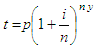# Future Value Calculator

Below you can find a future value calculator of an investment when the given interest is a factor to consider.

On this calculator, it's necessary to provide the amount of the initial investment, the nominal interest rate, the number of compounding periods per year and the number of years of the investment.

Assuming that there are no additional deposits or withdrawals, the future value of an investment is based on the folowing formula:where:

t = total value after y years (future value)
y = number of years
p = initial investment
i = nominal interest rate
n = number of compounding periods per year

We can get this task done with the following calculator...

### Example:

Mr. Karl M. makes a modest investment of \$100,000 at 9.5%. If interest is compounded quarterly, let's calculate the future value of Karl's investment in 15 years...

We enter our data into the calculator...

Initial Investment = 100,000.00
Nominal Interest rate = 9.50%
Nr. of Compounding periods  / yr =  4
Number of years = 15

and we get this result

Amount =     408,916.74

 Please allow JavaScript codes run on your navigator, otherwise this calculator won't work.

 Enter the needed values... (do not use commas) Initial Investment: Nominal Interest Rate: % Number of compounding periods/year: Number of years: Amount:

From 'Future Value Calculator' to home

From 'Future Value Calculator' to Free Online Calculators